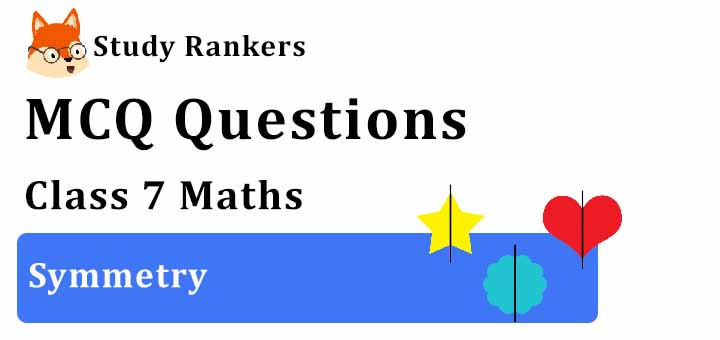## MCQ Questions for Class 7 Maths: Ch 14 Symmetry1. Which of the following letters have reflection line of symmetry about vertical mirror?
(a) C
(b) V
(c) B
(d) Q
► (b) V

2. How many lines of symmetries are there in regular pentagon?
(a) 2
(b) 3
(c) 5
(d) 4
► (c) 5

3. Number of lines of symmetry a triangle does not have:
(a) 3
(b) 1
(c) 0
(d) 2
► (d) 2

4. A In A XYZ, XY = XZ and XM⊥YZand ZP⊥XY. About which of the following is the triangle symmetrical?
(a) XM
(b) YN
(c) ZP
(d) XZ
► (a) XM

5. How many lines of symmetries are there in rectangle?
(a) 2
(b) 1
(c) 0
(d) None of these
► (a) 2

6. How many lines of symmetries are there in an equilateral triangle?
(a) 2
(b) 3
(c) 0
(d) 1
► (b) 3

7. Letter ‘C’ of the English alphabet have reflectional symmetry (i.e., symmetry related to mirror reflection) about.
(a) a horizontal mirror
(b) a vertical mirror
(c) both
(d) None of these
► (a) a horizontal mirror

8. Letter ‘H’ of the English alphabet have reflectional symmetry (i.e., symmetry related to mirror reflection) about.
(a) a vertical mirror
(b) Both horizontal nand veritcal
(c) a horizontal mirror
(d) Neither horizontal nor veritcal
► (b) Both horizontal nand veritcal

9. Which of the following triangles has no line of symmetry?
(a) An equilateral triangle
(b) An isosceles triangle
(c) A scalene triangle
(d) All of the above
► (c) A scalene triangle

10. Which of the following alphabets has line symmetry?
(a) P
(b) Q
(c) Z
(d) A
► (d) A

11. Which of the followings has both horizontal as well as vertical line of symmetry?
(a) H
(b) S
(c) V
(d) A
► (a) H

12. Which of the following alphabets has many lines of symmetry?
(a) I
(b) O
(c) P
(d) F
► (b) O

13. Which of these quadrilaterals have both line and rotational symmetries of order more than 3?
(a) A triangle
(b) A square
(c) A kite
(d) A rectangle
► (b) A square

14. Letter ‘D’ of the English alphabet have reflectional symmetry (i.e., symmetry related to mirror reflection) about.
(a) a vertical mirror
(b) a horizontal mirror
(c) both
(d) None of these
► (a) a vertical mirror

15. How many lines of symmetries are there in a square?
(a) 2
(b) 3
(c) 4
(d) 1
► (c) 4

16. Which of these letters has only rotational symmetry?
(a) S
(b) E
(c) B
(d) P
► (a) S

17. Letter ‘G’ of the English alphabet have reflectional symmetry (i.e., symmetry related to mirror reflection) about.
(a) Neither horizontal nor veritcal
(b) a horizontal mirror
(c) a vertical mirror
(d) both
► (a) Neither horizontal nor veritcal

18. What is the order of rotational symmetry of the English alphabet Z?
(a) 0
(b) 1
(c) 2
(d) 3
► (c) 2